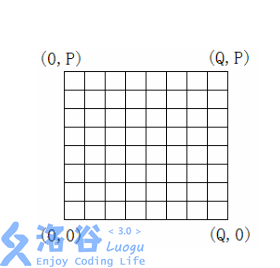P4012 深海机器人问题

• 461通过
• 1.1K提交
• 题目提供者 zzlzk
• 评测方式 云端评测
• 标签 图的建立,建图 最大流 网络流24题 O2优化
• 难度 省选/NOI-
• 时空限制 1000ms / 256MB
• 提示：收藏到任务计划后，可在首页查看。

题目描述

深海资源考察探险队的潜艇将到达深海的海底进行科学考察。

潜艇内有多个深海机器人。潜艇到达深海海底后，深海机器人将离开潜艇向预定目标移动。

深海机器人在移动中还必须沿途采集海底生物标本。沿途生物标本由最先遇到它的深海机器人完成采集。

每条预定路径上的生物标本的价值是已知的，而且生物标本只能被采集一次。

本题限定深海机器人只能从其出发位置沿着向北或向东的方向移动，而且多个深海机器人可以在同一时间占据同一位置。

用一个 $P\times Q$ 网格表示深海机器人的可移动位置。西南角的坐标为 $(0,0)$，东北角的坐标为 $(Q,P)$ 。给定每个深海机器人的出发位置和目标位置，以及每条网格边上生物标本的价值。

计算深海机器人的最优移动方案， 使深海机器人到达目的地后，采集到的生物标本的总价值最高。

输入输出格式

输入格式：

文件的第 $1$ 行为深海机器人的出发位置数 $a$,和目的地数 $b$ 。

第 $2$ 行为 $P$ 和 $Q$ 的值。

接下来的 $P+1$ 行,每行有 $Q$ 个正整数,表示向东移动路径上生物标本的价值,行数据依从南到北方向排列。

再接下来的 $Q+1$ 行,每行有 $P$ 个正整数,表示向北移动路径上生物标本的价值,行数据依从西到东方向排列。

接下来的 $a$ 行,每行有 $3$ 个正整数 $k,x,y$,表示有 $k$ 个深海机器人从 $(x,y)$ 位置坐标出发。

再接下来的 $b$ 行,每行有 $3$ 个正整数 $r,x,y$ ,表示有 $r$ 个深海机器人可选择 $(x,y)$ 位置坐标作为目的地。

a行和b行输入时横纵坐标要反过来

输出格式：

输出采集到的生物标本的最高总价值.

输入输出样例

输入样例#1： 复制
1 1
2 2
1 2
3 4
5 6
7 2
8 10
9 3
2 0 0
2 2 2
输出样例#1： 复制
42

说明

$1\leq P,Q\leq15$

$1\leq a\leq 4$

$1\leq b\leq 6$

提示
标程仅供做题后或实在无思路时参考。
请自觉、自律地使用该功能并请对自己的学习负责。
如果发现恶意抄袭标程，将按照I类违反进行处理。